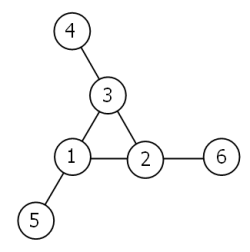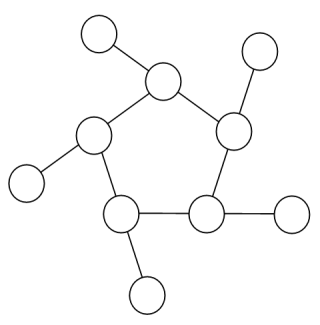## Project Euler – Problem 68

Problem 68:
Consider the following “magic” 3-gon ring, filled with the numbers 1 to 6, and each line adding to nine.Working clockwise, and starting from the group of three with the numerically lowest external node (4,3,2 in this example), each solution can be described uniquely. For example, the above solution can be described by the set: 4,3,2; 6,2,1; 5,1,3.

It is possible to complete the ring with four different totals: 9, 10, 11, and 12. There are eight solutions in total.

```Total	Solution Set
9	4,2,3; 5,3,1; 6,1,2
9	4,3,2; 6,2,1; 5,1,3
10	2,3,5; 4,5,1; 6,1,3
10	2,5,3; 6,3,1; 4,1,5
11	1,4,6; 3,6,2; 5,2,4
11	1,6,4; 5,4,2; 3,2,6
12	1,5,6; 2,6,4; 3,4,5
12	1,6,5; 3,5,4; 2,4,6```

By concatenating each group it is possible to form 9-digit strings; the maximum string for a 3-gon ring is 432621513.

Using the numbers 1 to 10, and depending on arrangements, it is possible to form 16- and 17-digit strings. What is the maximum 16-digit string for a “magic” 5-gon ring?``` class runner { private static void swap(byte[] set, int l, int k){ byte tmp = set[l]; set[l] = set[k]; set[k] = tmp; } public static boolean permute(byte[] store){ int k = -1; for(int i=0;i store[i]) l=i; } } swap(store,l,k); int diff = store.length - (k+1); for(int i=0; i 0){ max = cur; } } }while(permute(store)); System.out.println(max); System.out.println("time: "+(System.currentTimeMillis() - time)); } } ```

Note: Notice that you can wrap the inner circle of the 5-gon into an array…
Note2: Above code can be optimized by 1: Removing duplicates, 2. Assigning outer array 6-10 at the start.